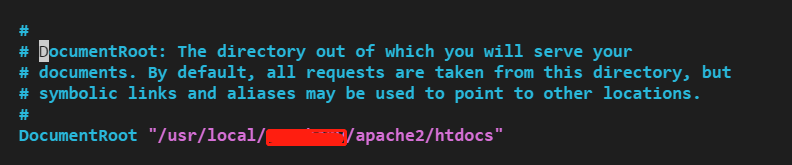# Apache与php快速部署web服务

[本文出自天外归云的博客园]<html>
<?php
$path =$_GET["path"];
$dir = "/cfs";$file_path = $dir.$path;
if (file_exists($file_path)) {$file_handle = fopen($file_path, "r"); while (!feof($file_handle)) {
$line = fgets($file_handle);
echo $line; echo '</br>'; } close($file_handle);
} else {
</html>